NEET  >  Test: Work, Energy & Power - 1

# Test: Work, Energy & Power - 1

Test Description

## 30 Questions MCQ Test Physics Class 11 | Test: Work, Energy & Power - 1

Test: Work, Energy & Power - 1 for NEET 2022 is part of Physics Class 11 preparation. The Test: Work, Energy & Power - 1 questions and answers have been prepared according to the NEET exam syllabus.The Test: Work, Energy & Power - 1 MCQs are made for NEET 2022 Exam. Find important definitions, questions, notes, meanings, examples, exercises, MCQs and online tests for Test: Work, Energy & Power - 1 below.
Solutions of Test: Work, Energy & Power - 1 questions in English are available as part of our Physics Class 11 for NEET & Test: Work, Energy & Power - 1 solutions in Hindi for Physics Class 11 course. Download more important topics, notes, lectures and mock test series for NEET Exam by signing up for free. Attempt Test: Work, Energy & Power - 1 | 30 questions in 30 minutes | Mock test for NEET preparation | Free important questions MCQ to study Physics Class 11 for NEET Exam | Download free PDF with solutions
 1 Crore+ students have signed up on EduRev. Have you?
Test: Work, Energy & Power - 1 - Question 1

### The scalar product of two vectors A and B is

Detailed Solution for Test: Work, Energy & Power - 1 - Question 1

Explanation:Scalar product means dot product and dot product of 2 vectors gives a scalar  , example  dot product of force and displacement gives work which is scalar

Test: Work, Energy & Power - 1 - Question 2

### Mass and energy are equivalent and are related by

Detailed Solution for Test: Work, Energy & Power - 1 - Question 2

Explanation:Mass–Energy equivalence states that anything having mass has an equivalent amount of energy and vice versa, with these fundamental quantities directly relating to one another by Einstein's theory of special relativity. It shows that matter (as mass) and energy can be converted into each other according to the famous equation E=mc2

Test: Work, Energy & Power - 1 - Question 3

### A railway porter is holding a weight of 100 kgs on his head and is standing still. What is the work done by him?

Detailed Solution for Test: Work, Energy & Power - 1 - Question 3

Explanation:W = Force x displacement in the direction of forceWork done will be zero because porter is stationary (i.e. displacement is zero)

Test: Work, Energy & Power - 1 - Question 4

A person trying to lose weight (dieter) lifts a 10 kg mass, one thousand times, to a height of 0.5 m each time. Assume that the potential energy lost each time she lowers the mass is dissipated. How much work does she do against the gravitational force?

Detailed Solution for Test: Work, Energy & Power - 1 - Question 4

Explanation:Work done by force applied against gravity for one lift will be

W = Fs = mgh = 10 x 9.8 x 0.5 = 49J

So work done for 1000 lifts = 49 x 1000 = 49000 J

Test: Work, Energy & Power - 1 - Question 5

A12-pack of Omni-Cola (mass 4.30 kg) is initially at rest on a horizontal floor. It is then pushed in a straight line for 1.20 m by a trained dog that exerts a horizontal force with magnitude 36.0 N. Use the work–energy theorem to find the final speed of the 12-pack if there is no friction between the 12-pack and the floor

Detailed Solution for Test: Work, Energy & Power - 1 - Question 5

Explanation:

from work–energy theorem

change in kinetic energy = work done

ΔK = W

Kf - Ki = Fs

1/2mv2 - 0 = Fs

m = 4.30 kg, s = 1.2m, F = 36N

vf = vm/s , vi = 0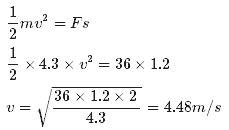Test: Work, Energy & Power - 1 - Question 6

The work done by the force is defined to be

Detailed Solution for Test: Work, Energy & Power - 1 - Question 6

Explanation:Work done is given by

W = (Fcosθ)d

here Fcosθ is the component of applied force  in direction of displacement and d is magnitude of displacement.

Test: Work, Energy & Power - 1 - Question 7

Power is defined as

Detailed Solution for Test: Work, Energy & Power - 1 - Question 7

Explanation:Power is the rate of doing work. It is the amount of energy consumed per unit time. Having no direction, it is a scalar quantity. In the SI system, the unit of power is the joule per second (J/s), known as the watt.The integral of power over time defines the work performed.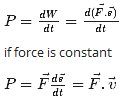Test: Work, Energy & Power - 1 - Question 8

A weight of 20kg falls from a height of 10 m. The work done by the gravitational force is (Take g = 10 mms-2)

Detailed Solution for Test: Work, Energy & Power - 1 - Question 8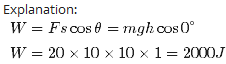Test: Work, Energy & Power - 1 - Question 9

S.I unit of power is

Detailed Solution for Test: Work, Energy & Power - 1 - Question 9

Explanation:The SI unit of power, equivalent to one joule per second, corresponding to the rate of consumption of energy (Work done).

Test: Work, Energy & Power - 1 - Question 10

In which case is the work done zero?

Detailed Solution for Test: Work, Energy & Power - 1 - Question 10

Explanation:Work done is given as

W = Fdcosθ

Here θ is the angle between F and d  if both are perpendicular  then θ = 90 degree so cosθ = 0  and thus work done is 0 .

Test: Work, Energy & Power - 1 - Question 11

Which of the following laws used to solve problems in collisions?

Detailed Solution for Test: Work, Energy & Power - 1 - Question 11

Explanation:If external force is absent then linear momentum of system remains conserved. In collision force of impact due to collision is internal and very large.

Test: Work, Energy & Power - 1 - Question 12

A pump on the ground floor of a building can pump up water to fill a tank of volume 30 m3 in 15 min. If the tank is 40 m above the ground, and the efficiency of the pump is 30%,  how much electric power is consumed by the pump?

Detailed Solution for Test: Work, Energy & Power - 1 - Question 12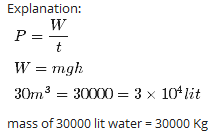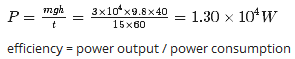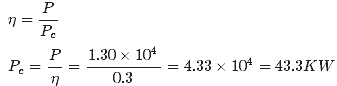Test: Work, Energy & Power - 1 - Question 13

The S.I unit of force is

Detailed Solution for Test: Work, Energy & Power - 1 - Question 13

Explanation:In International System of Units (SI) the newton is the unit for force. It is equal to the amount of net force required to accelerate a mass of one kilogram at a rate of 1 m/sec2 in direction of the applied force. It is named after Isaac Newton in recognition of his work on classical mechanics, specifically Newton's second law of motion.

1N=1Kgm/sec2

Dyne is a  cgs unit of force. One dyne is equal to 10−5 N

Test: Work, Energy & Power - 1 - Question 14

In an elastic collision

Detailed Solution for Test: Work, Energy & Power - 1 - Question 14

Explanation:kinetic energy is transferred to other forms of energy—such as thermal energy, potential energy, and sound—during the collision process. After collision if recovery of kinetic energy is 100% then it is called elastic collision.So that in an elastic collision the total kinetic energy of the system is the same after the collision as before.

Test: Work, Energy & Power - 1 - Question 15

Work-energy theorem does not give information on

Detailed Solution for Test: Work, Energy & Power - 1 - Question 15

Explanation:According to work energy theorem :Net work done on a body equals change in its kinetic energySo it does not give any information about time dependence.

Test: Work, Energy & Power - 1 - Question 16

In an inelastic collision

Detailed Solution for Test: Work, Energy & Power - 1 - Question 16

Explanation:kinetic energy is transferred to other forms of energy—such as thermal energy, potential energy, and sound—during the collision process. After collision if recovery of kinetic energy is less than % then it is called inelastic collision i.e.. some part of kinetic energy is not recover. So that in an inelastic collision the total kinetic energy after the collision is less than before the collision

Test: Work, Energy & Power - 1 - Question 17

Physically, the notion of potential energy is applicable only to

Detailed Solution for Test: Work, Energy & Power - 1 - Question 17

Explanation:Potential energy is the stored energy of an object. It is the energy by virtue of an object's position relative to other objects. Potential energy is often associated with restoring forces such as a spring or the force of gravity. It is applicable only for conservative forces.

Test: Work, Energy & Power - 1 - Question 18

If a machine is lubricated with oil

Detailed Solution for Test: Work, Energy & Power - 1 - Question 18

When a machine is lubricated with oil friction decreases. Hence the mechanical efficiency of the machine increases.

Test: Work, Energy & Power - 1 - Question 19

A bolt of mass 0.3 kg falls from the ceiling of an elevator moving down with an uniform speed of 7 m/s. It hits the floor of the elevator (length of the elevator = 3 m) and does not rebound. What is the heat produced by the impact?

Detailed Solution for Test: Work, Energy & Power - 1 - Question 19

Explanation:

Whole of the potential energy of bolt converted in to heat energy

heat produced by the impact = mgh =0.3×9.8×3=8.82J

Test: Work, Energy & Power - 1 - Question 20

The work done by a conservative force

Detailed Solution for Test: Work, Energy & Power - 1 - Question 20

Explanation:A force is said to be conservative if work done by this force is independent of path and is dependent only on end points .

Test: Work, Energy & Power - 1 - Question 21

In which of the following cases is the work done positive?

Detailed Solution for Test: Work, Energy & Power - 1 - Question 21

Explanation:Work done is positive when force applied and displacement are in same direction.

W=F .s =Fscosθ

in this case angle between force and displacement is zero (i.e. cosθ=1) so that work done is positive.

Test: Work, Energy & Power - 1 - Question 22

The total mechanical energy of a system is conserved if the

Detailed Solution for Test: Work, Energy & Power - 1 - Question 22

Explanation:Mechanical energy is the sum of kinetic and potential energy in an object that is used to do work. In other words, it is energy in an object due to its motion or position, or both. In case of conservative forces total mechanical energy remains conserved because potential energy applicable only for conservative forces.

Test: Work, Energy & Power - 1 - Question 23

The casing of a rocket in flight burns up due to friction. At whose expense is the heat energy required for burning obtained?

Detailed Solution for Test: Work, Energy & Power - 1 - Question 23

Explanation:Heat energy required for burning of casing of rocket comes from the rocket itself. As a result of work done against friction the kinetic energy of rocket continuously deceases and this work against friction reappears as heat energy. This results in reduction of the mass of the rocket.

Test: Work, Energy & Power - 1 - Question 24

A tandem (two-person) bicycle team must overcome a force of 165 N to maintain a speed of 9.00 m/s. Find the power required per rider, assuming that each contributes equally.

Detailed Solution for Test: Work, Energy & Power - 1 - Question 24

Explanation:

Total power required to overcome a force of 165 N and to maintain a speed of 9.00 m/s

F = 165N

v = 9m/s

P = Fv = 165 x 9 = 1485 W

if each rider contribute equal power, then power required per rider will be P/2 = 1485/2 = 742.5W

Test: Work, Energy & Power - 1 - Question 25

For a ball dropped from a tower of height h the total mechanical energy is

Detailed Solution for Test: Work, Energy & Power - 1 - Question 25

Explanation:mechanical energy = sum of potential and kinetic energiesa falling ball will have both these energies in between topmost and bottomost points of its motion so mechanical energy is the sum of potential and kinetic energies.

Test: Work, Energy & Power - 1 - Question 26

A trolley of mass 200 kg moves with a uniform speed of 36 km/h on a frictionless track. A child of mass 20 kg runs on the trolley from one end to the other (10 m away) with a speed of 4 ms−1 relative to the trolley in a direction opposite to the its motion, and jumps out of the trolley. How much has the trolley moved from the time the child begins to run?

Detailed Solution for Test: Work, Energy & Power - 1 - Question 26

Explanation:
Mass of troly M = 200Kg
mass of child m = 20Kg
speed of trolley v = 36Km/hr=36 x 5/18 = 10m/s
Let v' be the final velocity of the trolley with respect to the ground.
Final velocity of the boy with respect to the ground = v,−4
from conservation of linear momentum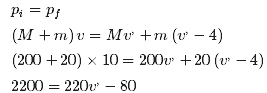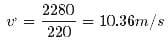Time taken by the boy to run t = 10/4 = 2.5 sec
Distance moved by the trolley = vt = 10.36 x 2.5 = 25.9 m

Test: Work, Energy & Power - 1 - Question 27

A 50.0-kg marathon runner runs up the stairs to the top of a 443-m-tall Tower. To lift herself to the top in 15.0 minutes, what must be her average power output?

Detailed Solution for Test: Work, Energy & Power - 1 - Question 27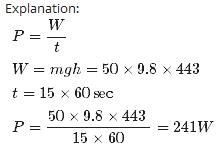Test: Work, Energy & Power - 1 - Question 28

In which of the following cases is the work done positive?

Detailed Solution for Test: Work, Energy & Power - 1 - Question 28

Explanation:When a body is moving on a rough horizontal surface then their will be 2 forces acting on the body   1. Applied force ( in the direction of motion) 2. friction ( opposite to direction of motion)As applied force is in same direction as displacement so work done will be positive.

Test: Work, Energy & Power - 1 - Question 29

The launching mechanism of a toy gun consists of a spring of unknown spring constant. When the spring is compressed 0.120 m, the gun, when fired vertically, is able to launch a 35.0-g projectile to a maximum height of 20.0 m above the position of the projectile before firing. Neglecting all resistive forces, determine the spring constant.

Detailed Solution for Test: Work, Energy & Power - 1 - Question 29

Explanation:

Potential energy of spring converted in to potential energy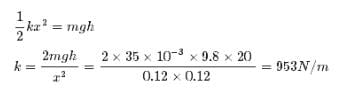Test: Work, Energy & Power - 1 - Question 30

If F is a force and d is the displacement in the direction of force then the work done by the force is given by

Detailed Solution for Test: Work, Energy & Power - 1 - Question 30

Explanation:Work done = force in the direction of displacement multiplied by displacement

W=F.d

## Physics Class 11

126 videos|449 docs|210 tests
 Use Code STAYHOME200 and get INR 200 additional OFF Use Coupon Code
Information about Test: Work, Energy & Power - 1 Page
In this test you can find the Exam questions for Test: Work, Energy & Power - 1 solved & explained in the simplest way possible. Besides giving Questions and answers for Test: Work, Energy & Power - 1, EduRev gives you an ample number of Online tests for practice

## Physics Class 11

126 videos|449 docs|210 tests

### How to Prepare for NEET

Read our guide to prepare for NEET which is created by Toppers & the best Teachers